# Java Operators in 2022: The Complete Guide

0
7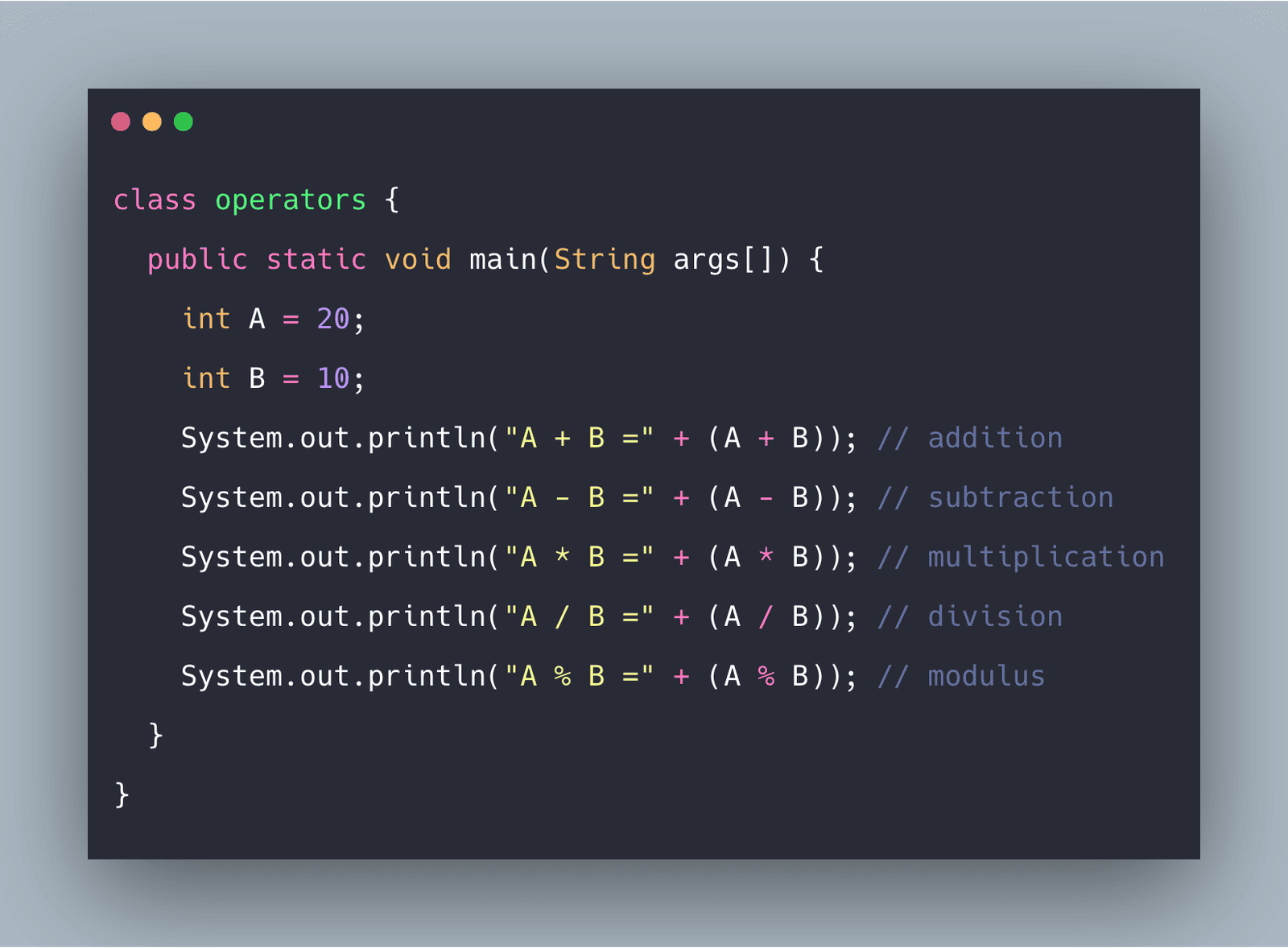Java operators are the symbols in java that are used to perform an operation on variable and their values.

## Java Operators

Operators in Java are used to performing operations on variables and values. A value is called an operand, while the operation (to be performed between the two operands) is defined by an operator.

There are the following types of Operators In Java.

1. Arithmetic Operators
2. Relational Operators
3. Logical Operator
4. Assignment Operators
5. Bitwise Operators
6. Unary Operator
7. Ternary Operators
8. Shift Operators

## Arithmetic Operators

Arithmetic operators are used to performing simple arithmetic operations like addition, subtraction, etc.

 Operators Name Example  (A=20, B=10) Result + Addition A+B 30 – Subtraction A-B 10 * Multiplication A*B 200 / Division A/B 2 % Modulus A%B 0

See the following program.

```class operators {
public static void main(String args[]) {
int A = 20;
int B = 10;
System.out.println("A + B =" + (A + B)); // addition
System.out.println("A - B =" + (A - B)); // subtraction
System.out.println("A * B =" + (A * B)); // multiplication
System.out.println("A / B =" + (A / B)); // division
System.out.println("A % B =" + (A % B)); // modulus
}
}```

See the following output.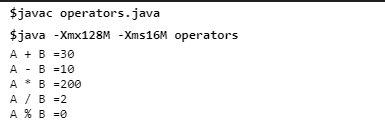## Relational Operators

Relational operators are used to comparing the values. They return true or false as an output.

 Operators Name Example  (A=20, B=10) Result < Less than A Greater than A>B true >= Greater than or equal to A>=B true == Equal to A==B false != Not equal A!=B true

See the following program.

```class relation {
public static void main(String args[]) {
int A = 20;
int B = 10;
System.out.println("A < B:" + (A < B)); // less than
System.out.println("A <= B:" + (A <= B)); // less than equal to
System.out.println("A > B:" + (A > B)); // greater than
System.out.println("A >= B:" + (A >= B)); // greater tahn equal to
System.out.println("A == B:" + (A == B)); // equal to
System.out.println("A != B=" + (A != B)); // not equal
}
}```

See the following code.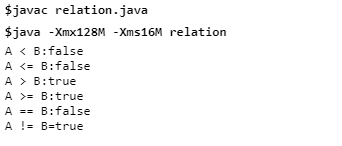## Logical operators

These operators are used to perform some special operations like logical AND, logical OR etc. It also returns the output as true and false.

 Operators Name Example (A=20,B=10) Result && Logical AND A<30&&B>5 true || Logical OR A>10||B<5 true ! Logical NOT !(A>=B) false

See the following program.

```class logical {
public static void main(String args[]) {
int A = 20;
int B = 10;
System.out.println("A && B =" + (A < 30 && B > 5)); // true if both are true
System.out.println("A || B =" + (A > 10 || B < 5)); // true if one is true
System.out.println("A ! B =" + (!(A > B))); // reverse the output
}
}```

See the following output.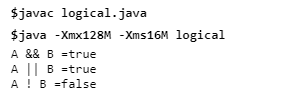## Assignment Operator

An assignment operator is used to assign a value to the variable. The (=) is called an assignment operator in Java.

 Operators Example  A=20,B=10 Result A=B Assign the value of B to A 10 += A+=B 30 -= A-=B 10 *= A*=B 200 /= A/=B 2 %= A%=B 0

## Bitwise Operator

These operators are used for manipulating individual bits of the operand.

Some of the bitwise operators are:

1. Bitwise AND (&)
2. Bitwise OR (|)
3. Bitwise NOT (~)
4. Bitwise XOR

See the following code.

```public class bitwise {
public static void main(String args[]) {
int A = 0x014;
int B = 0x016;
System.out.println("A & B=" + (A & B));
System.out.println("A | B=" + (A | B));
System.out.println(" ~A =" + (~A));
}
}```

See the following output.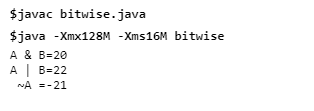## Unary Operator

These operators are used where only one operand is required.

Various types of unary operators are the following.

### Increment operator

It is used to increment/increase the value by 1. It is again to two types

1. pre-increment: at first, the value is incremented the transferred to the output.
2. post-increment: at first it gives the output then the value is incremented.

### Decrement operator

It is used to decrement/decrease the value by 1. It is also of two types.

1. pre-decrement: at first the value is decremented then output is shown
2. post-decrement: at first it gives the result then the value is decremented.
3. !- logical not used for inverting the values of Boolean.

See the following program.

```class unary {
public static void main(String args[]) {
int A = 40;
System.out.println(A++);
System.out.println(++A);
System.out.println(A--);
System.out.println(--A);
}
}```

See the following output.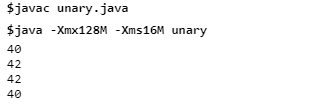## Ternary Operator

It is the short version of the if-else statement which is written in one line.

### Syntax

```Condition? if true:  if false
```

See the following program.

```class ternary {
public static void main(String args[]) {
int x = 20;
int y = 10;
int gre = (x > y) ? x : y;
System.out.println(gre);
}
}```

See the following output.

##Shift operator in Java

The shift operator is used to shift the bit of number in terms of multiplying and dividing by 2^n.

It is of two types:

1. Left shift (<<): In this, the value in bits shift towards the left side which means the number is multiplied by 2^n.
2. Right shift (>>): In this, the value in bits shift toward the right side which means the number is divided by 2^n.

See the following program.

```class shift {
public static void main(String args[]) {
System.out.println(4 << 2); // 4*2^n : 4*2^2=16
System.out.println(4 << 3); // 4*2^3=32
System.out.println(4 >> 2); // 4/2^2=1
System.out.println(16 >> 3); // 16/2^3=2
}
}```

See the following output.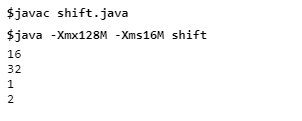That’s it for this tutorial.

This site uses Akismet to reduce spam. Learn how your comment data is processed.